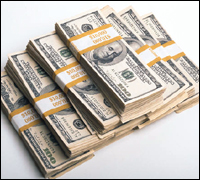$MONEY$

Algebra Level 1If $80 is divided among three people so that the second person will have twice as much as the first, and the third person will have$5 less than the second, then how many dollars will the third person have?

×